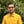Trusted answers to developer questions
Trusted Answers to Developer Questions

Related Tags

d
math
communitycreator

# What is the isFinite() function in D?Harris Amjad

Grokking Modern System Design Interview for Engineers & Managers

Ace your System Design Interview and take your career to the next level. Learn to handle the design of applications like Netflix, Quora, Facebook, Uber, and many more in a 45-min interview. Learn the RESHADED framework for architecting web-scale applications by determining requirements, constraints, and assumptions before diving into a step-by-step design process.

### Overview

A number that can be counted is called a finite number. The isFinite() function is used to check if the number is finite.

The figure below shows the visual representation of the isFinite() function:

Visual representation of isFinite() function

Note: Import std.math in your code to use the isFinite() function. We can import it:
import std.math

### Syntax

bool isFinite(number)


### Parameter

This function requires the number as a parameter.

### Return value

This function returns true if the value is finite. Otherwise, it returns false.

Note: NaN is not considered to be finite.

### Example

The following example shows how to use isFinite() in D:

import core.stdc.stdio;import std.stdio;//header required for functionimport std.math;int main(){    //integer    writeln ("The value of isFinite(4.0) : ",isFinite(4.0));    //positive double value    writeln ("The value of isFinite(2.56) : ",isFinite(2.56));    //negative double value    writeln ("The value of isFinite(-2.56) : ",isFinite(-2.56));    //zero    writeln ("The value of isFinite(0.0) : ",isFinite(0.0));    //infinity    writeln ("The value of isFinite(real.infinity) : ",isFinite(real.infinity));    writeln ("The value of isFinite(-real.infinity) : ",isFinite(-real.infinity));        //nan    writeln ("The value of isFinite(real.nan) : ",isFinite(real.nan));    writeln ("The value of isFinite(-real.nan) : ",isFinite(-real.nan));    return 0;}
using the isFinite() method in D

### Explanation

Here is a line-by-line explanation of the code above:

• Line 4: We add std.math library which is required to use isFinite() function.
• Line 9: We check if the integer 4 is finite or not using the isFinite() function.
• Line 12: We check if the positive double value 2.56 is finite or not using the isFinite() function.
• Line 15: We check if the negative double value -2.56 is finite or not using the isFinite() function.
• Line 18: We check if the zero is finite or not using the isFinite() function.
• Lines 21 to 22: We check if the positive infinity and negative infinity are finite or not using the isFinite() function.
• Lines 25 to 26: We check if the positive NaN and negative NaN are finite or not using the isFinite() function.

RELATED TAGS

d
math
communitycreator

CONTRIBUTORHarris Amjad

Grokking Modern System Design Interview for Engineers & Managers

Ace your System Design Interview and take your career to the next level. Learn to handle the design of applications like Netflix, Quora, Facebook, Uber, and many more in a 45-min interview. Learn the RESHADED framework for architecting web-scale applications by determining requirements, constraints, and assumptions before diving into a step-by-step design process.

Keep Exploring

Learn in-demand tech skills in half the time